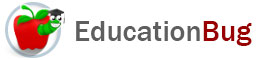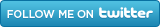PUBLIC SCHOOLS PRIVATE SCHOOLS SCHOOL DISTRICTS COLLEGES PUBLIC LIBRARIES JOBS BLOG RESOURCES
Career Education
Education Choices
Education Costs
Education History and General Information
Education Issues
Education Resources
Family Involvement in Education
Students w Special Needs
Subject Information and Homework Help
Teaching and Learning
Testing
By Types of Schools
By Specific Audiences
GeneralRoman Numerals

Roman numerals are symbols that represent numbers. Read this article for more information on how Roman numerals are organized and used. Also, see a chart showing the Roman numerals from one to one million.

Sometimes people thing of the numerals that we characteristically use (0, 1, 2, 3, 4, 5, 6, 7, 8, 9) as the system, but it is only one of a number of systems of mathematical notation devised to represent numbers and operations using numbers. The name of the system using the ten familiar digits is the Arabic numerals. This article explains an alternative system based on a system devised in Ancient Rome. It is called the Roman numerals. For more about Roman numerals, keep reading.

The Symbols Used in Roman Numerals

The Roman numeral system uses symbols that we are more familiar with as letters. They are as follows:

 Number Name Arabic Numeral Roman Numeral Roman Numeral small zero 0 none none one 1 I i five 5 V v ten 10 X x fifty 50 L l one hundred 100 C c five hundred 500 D d one thousand 1,000 M m five thousand 5,000 VÌ… vÌ… ten thousand 10,000 CÌ… cÌ… one hundred thousand 100,000 DÌ… dÌ… one million 1,000,000 MÌ… mÌ…

There are several important difference to note so far:

• The Roman numerals system has no zero.
• The Arabic numerals system represents all of the twelve numbers shown with three symbols: 0, 1, 5. (A comma is also used, but the numbers would still be interpretable without them.) The Roman numerals canâ€™t express one of the numbers and use 11 different symbols to express the other eleven.

Making Numbers with Roman Numerals

So, given how differently theyâ€™re configured compared with Arabic numerals, you may be wondering just how Roman numerals work. There are a couple of major rules for building numbers. Weâ€™ll present them in comparison with how things are done with Arabic numerals, for comparison.

• Arabic numerals represent a digit by a single symbol: 0, 1, 2, 3, 4, 5, 6, 7, 8, or 9. Roman numerals represent a digit by up to three symbols:

Identical symbols can be added by being placed side by side:

• a single symbol can represent a digit, as in I for one
• two symbols can represent a digit as in II for two
• three symbols can represent a digit as in III for three

Different symbols can be added by up to three instances of the smaller one being placed to the right of the larger one:

• VI is six
• VII is seven
• VIII is eight

Different symbols can be subtracted by one instance of the smaller one being place to the left of the larger one, but there is a limit to which symbols can be used, with the allowable symbols for up to a thousand being show in the following list:

• IV is four
• IX is nine
• XL is forty
• XC is ninety
• CD is four hundred
• CM is nine hundred

Both Roman numerals and Arabic numerals place the largest amount to the left.

• In Arabic numerals, this is conceived in digits, and the value of the digit is represented by its position in the framework of tens, hundreds, thousands, ten thousands, hundred thousands, millions, etc., and zeroes are included.
• In Roman numerals, the value of the largest amount is represented by one or more symbols placed on the left, followed by the next amount, and the next - but places that would be represented by zeroes in the Arabic numeral system are skipped.

Thus, to write one million, the Arabic numeral system requires a place holder for the hundred thousands, the ten thousands, the thousands, the hundreds, the tens, and the ones, whereas the Roman numeral system conveys the number with a single symbol: MÌ…

In such instances, Roman numerals are easier to grasp at a glance than Arabic numerals.

Working with Roman Numerals

Because the Roman numerals are not aligned in places, as Arabic numerals are, they do not have the same ease of use for doing operations that Arabic numerals do.

349

438+

in Arabic numerals, looks like this in Roman numerals:

CCCXVIX

CDXXXVIII

Notice that the number of symbols used to make the two numbers we want to add are the same in Arabic numerals and different in Roman numerals. Nevertheless, at first glance, it might look as if you could just put, for example, all the Câ€™s together and add them, but since the first C in 438 is actually subtracting value from the D, this isnâ€™t possible. This one example demonstrates that doing operations with Roman numerals could be far more time consuming than with Arabic numerals.